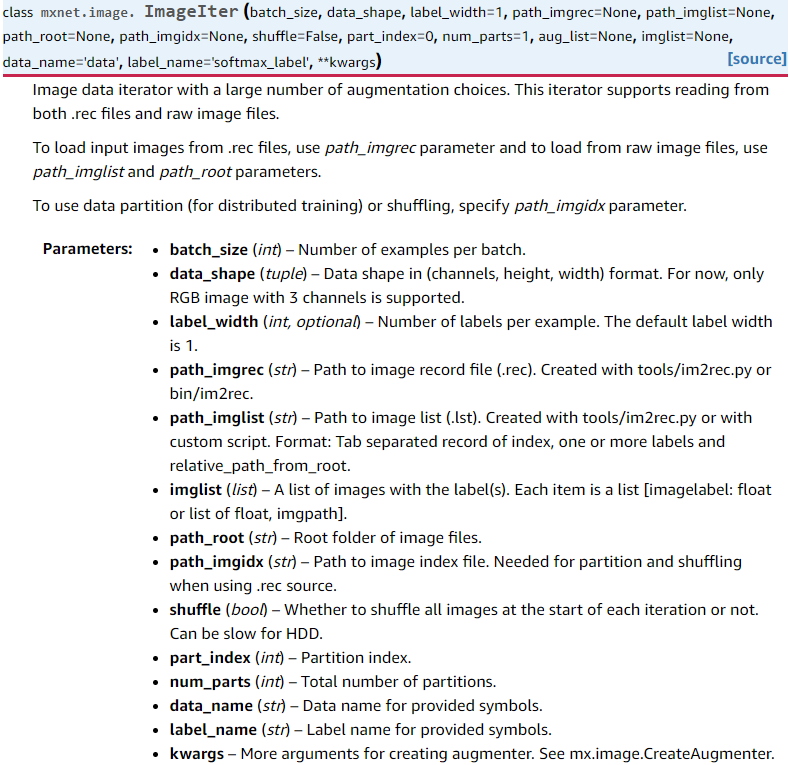# MXNet 导入图像数据

MXNet框架用于做图像相关的项目时，读取图像主要有两种方式：第一种是读.rec格式的文件，类似Caffe框架中LMDB，优点是.rec文件比较稳定，移植到别的电脑上也能复现，缺点是占空间（.rec文件的大小基本上和图像的存储大小差不多），而且增删数据不大灵活。第二种是.lst和图像结合的方式，首先在前面生成.rec文件的过程中也会生成.lst文件，这个.lst文件就是图像路径和标签的对应列表，也就是说通过维护这个列表来控制你训练集和测试集的变化，优点是灵活且不占空间，缺点是如果图像格式不符合要求的话容易出错而且如果列表中的某些图像路径对应的图像文件夹中图像被删除，就寻找不到，另外如果你不是从固态硬盘上读取图像的话，速度会很慢。

MXNet的图像数据导入模块主要有mxnet.io.ImageRecordItermxnet.image.ImageIter两个类，前者主要用来读取.rec格式的数据，后者既可以读.rec格式文件，也可以读原图像数据。脚本image.py可以在~/mxnet/python/mxnet/image.py找到.

#### 1.1、生成.lst

python ~/incubator-mxnet/tools/im2rec.py --list True --recursive True --train-ratio 0.9 /home/lst/data /home/image
or
os.system('python %s/tools/im2rec.py --list=1 --recursive=1 --shuffle=1 --test-ratio=0.2 data/caltech data/101_ObjectCategories'%os.environ['MXNET_HOME'])
–list参数必须要是True，说明你是要生成.lst文件，–recursive参数必须为True，表示要将所有图像路径写进成.lst文件，–train-ratio参数表示将train和val以多少比例划分，默认为1，表示都是train的数据。

.lst文件样例：第一列是index，第二列是label，第三列是图像路径

#### 1.2、生成.rec

python ~/incubator-mxnet/tools/im2rec.py --num-thread 4 /home/lst /home/image
or
os.system("python %s/tools/im2rec.py --num-thread=4 --pass-through=1 data/caltech data/101_ObjectCategories"%os.environ['MXNET_HOME'])

mx.io.ImageRecordIter(
path_imgrec         = args.data_train,
path_imgidx         = args.data_train_idx,
label_width         = 1,
mean_r              = rgb_mean,
mean_g              = rgb_mean,
mean_b              = rgb_mean,
data_name           = 'data',
label_name          = 'softmax_label',
data_shape          = image_shape,
batch_size          = args.batch_size,
rand_crop           = args.random_crop,
max_random_scale    = args.max_random_scale,
fill_value          = 127,
min_random_scale    = args.min_random_scale,
max_aspect_ratio    = args.max_random_aspect_ratio,
random_h            = args.max_random_h,
random_s            = args.max_random_s,
random_l            = args.max_random_l,
max_rotate_angle    = args.max_random_rotate_angle,
max_shear_ratio     = args.max_random_shear_ratio,
rand_mirror         = args.random_mirror,
shuffle             = True,
num_parts           = nworker,
part_index          = rank)

#### 2、.lst + 图像

mxnet.image.ImageIter是一个非常重要的类。在MXNet中，当你要读入图像数据时，可以用im2rec.py生成lst和rec文件，然后用mxnet.io.ImageRecordIter类来读取rec文件或者用这个mxnet.image.ImageIter类来读取rec文件，但是这个函数和前者相比还能直接读取图像文件，这样就可以不用生成占内存的rec文件了，只需要原图像文件和lst文件即可。另外，在mxnet.io.ImageRecordIter中对于数据的预处理操作都是固定的，不好修改，但是mxnet.image.ImageIter却可以非常灵活地添加各种预处理操作。接下来看看这个类。

### mxnet.image.ImageIterdata_iter = mx.image.ImageIter(batch_size=4, data_shape=(3, 227, 227),
path_imgrec="./data/caltech.rec",
path_imgidx="./data/caltech.idx" )

# data_iter的类型是mxnet.image.ImageIter
#reset()函数的作用是：resents the iterator to the beginning of the data
data_iter.reset()

#batch的类型是mxnet.io.DataBatch，因为next()方法的返回值就是DataBatch
batch = data_iter.next()

#data是一个NDArray，表示第一个batch中的数据，因为这里的batch_size大小是4，所以data的size是4*3*227*227
data = batch.data

#这个for循环就是读取这个batch中的每张图像并显示
for i in range(4):
plt.subplot(1,4,i+1)
plt.imshow(data[i].asnumpy().astype(np.uint8).transpose((1,2,0)))
plt.show()

mxnet.image.ImageIter中要灵活添加预处理可以通过mxnet.image.CreateAugmenter()函数，这个函数完整的定义是这样的：
mxnet.image.CreateAugmenter(data_shape, resize=0, rand_crop=False, rand_resize=False, rand_mirror=False, mean=None, std=None, brightness=0, contrast=0, saturation=0, pca_noise=0, inter_method=2)

train = mx.io.ImageRecordIter(
path_imgrec         = args.data_train,
label_width         = 1,
mean_r              = rgb_mean,
mean_g              = rgb_mean,
mean_b              = rgb_mean,
data_name           = 'data',
label_name          = 'softmax_label',
data_shape          = image_shape,
batch_size          = args.batch_size,
rand_crop           = args.random_crop,
max_random_scale    = args.max_random_scale,
fill_value          = 127,
min_random_scale    = args.min_random_scale,
max_aspect_ratio    = args.max_random_aspect_ratio,
random_h            = args.max_random_h,
random_s            = args.max_random_s,
random_l            = args.max_random_l,
max_rotate_angle    = args.max_random_rotate_angle,
max_shear_ratio     = args.max_random_shear_ratio,
rand_mirror         = args.random_mirror,
shuffle             = True,
num_parts           = nworker,
part_index          = rank)

train = mx.image.ImageIter(
batch_size            = args.batch_size,
data_shape          = (3,224,224),
label_width           = 1,
path_imglist          = args.data_train,
path_root              = args.image_train,
part_index            = rank,
shuffle                  = True,
data_name           = 'data',
label_name           = 'softmax_label',
aug_list                 = mx.image.CreateAugmenter((3,224,224),resize=224,rand_crop=True,rand_mirror=True,mean=True))

val部分可以类似修改，这里最重要的就是最后一个参数aug_list，表示所有预处理的列表，不过在val中一般不会有类似crop，mirror等操作。为什么会用到aug_list这个参数呢？来自于image.py脚本中ImageIter类的init()函数的这几行代码：

if aug_list is None:
self.auglist = CreateAugmenter(data_shape, **kwargs)
else:
self.auglist = aug_list